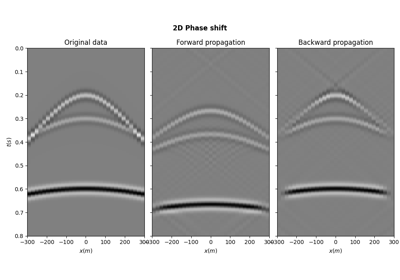# pylops.waveeqprocessing.PhaseShift#

pylops.waveeqprocessing.PhaseShift(vel, dz, nt, freq, kx, ky=None, dtype='float64', name='P')[source]#

Phase shift operator

Apply positive (forward) phase shift with constant velocity in forward mode, and negative (backward) phase shift with constant velocity in adjoint mode. Input model and data should be 2- or 3-dimensional arrays in time-space domain of size $$[n_t \times n_x \;(\times n_y)]$$.

Parameters
velfloat, optional

Constant propagation velocity

dzfloat, optional

Depth step

ntint, optional

Number of time samples of model and data

freqnumpy.ndarray

Positive frequency axis

kxint, optional

Horizontal wavenumber axis (centered around 0) of size $$[n_x \times 1]$$.

kyint, optional

Second horizontal wavenumber axis for 3d phase shift (centered around 0) of size $$[n_y \times 1]$$.

dtypestr, optional

Type of elements in input array

namestr, optional

New in version 2.0.0.

Name of operator (to be used by pylops.utils.describe.describe)

Returns
Poppylops.LinearOperator

Phase shift operator

Notes

The phase shift operator implements a one-way wave equation forward propagation in frequency-wavenumber domain by applying the following transformation to the input model:

$d(f, k_x, k_y) = m(f, k_x, k_y) e^{-j \Delta z \sqrt{\omega^2/v^2 - k_x^2 - k_y^2}}$

where $$v$$ is the constant propagation velocity and $$\Delta z$$ is the propagation depth. In adjoint mode, the data is propagated backward using the following transformation:

$m(f, k_x, k_y) = d(f, k_x, k_y) e^{j \Delta z \sqrt{\omega^2/v^2 - k_x^2 - k_y^2}}$

Effectively, the input model and data are assumed to be in time-space domain and forward Fourier transform is applied to both dimensions, leading to the following operator:

$\mathbf{d} = \mathbf{F}^H_t \mathbf{F}^H_x \mathbf{P} \mathbf{F}_x \mathbf{F}_t \mathbf{m}$

where $$\mathbf{P}$$ perfoms the phase-shift as discussed above.

## Examples using pylops.waveeqprocessing.PhaseShift#PhaseShift operator

PhaseShift operator